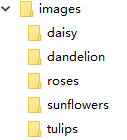• 1.%% 求解单变量方程%-----例子1------syms xeqn=sin(x)==1;...[solx,params,conds]=solve(eqn,x,'ReturnConditions',true)%-----例子3---------------%如果返回empty，则表明解不存在。如果返回emp...
1.%% 求解单变量方程%-----例子1------syms xeqn=sin(x)==1;solve(eqn,x)%-----例子2------syms xeqn=sin(x)==1;[solx,params,conds]=solve(eqn,x,'ReturnConditions',true)%-----例子3---------------%如果返回empty，则表明解不存在。如果返回empty+warning，则解可能存在，但是solve找不到syms xsolve(3*x+2,3*x+1,x)2.%% 求解多变量方程%---例1-----------------%为了避免求解方程时对符号参数产生混乱，需要指明一个等式中需要求解的变量。%如果不指明的话，solve函数就会通过symvar选择一个变量(认为该变量是要求解的变量)clc,clearsyms a b c xsola=solve(a*x^2+b*x+c==0,a)   %待求解的变量是asol=solve(a*x^2+b*x+c==0)  %待求解的变量是x%----例子2--------------%当求解的变量大于1个时，你声明变量的顺序就是slove返回解的顺序syms a b[b,a]=solve(a+b==1,2*a-b==4,b,a)3.%% solve返回的解带有：参数&条件%为了返回一个方程的完整的解(即解中含有的参数，及对参数的限制)，需要指定ReturnConditions 为：true%---例子1：关于解的约束----clc,clearsyms xS=solve(sin(x)==0 ,x,'ReturnConditions',true);SS.xS.parametersS.conditions%为了找到x的数值解，以一个值(利用函数subs)代替k。用函数isAlways检验该值是否满足关于k的限制%检验k=4是否满足in(k, 'integer')isAlways(subs(S.conditions,S.parameters,4))%isAlways返回的是logical 1(true)，这意味着：对于k而言，4是一个合法值。%利用4代替k，得到x的一个解。利用函数vpa获得该逼近的数值解(vpa设置数值精度：保留几位有效数字、几位小数)solx=subs(S.x,S.parameters,4)vpa(solx)%为0assume(S.conditions)solk=solve(S.x>0,S.x<2*pi,S.parameters)solx=subs(S.x,S.parameters,solk)4.%% 求解方程组(为变量分配解)------------%当求解方程组的时候，利用多个输出项对应求解的输出变量。%solve返回一个符号数组(为每个相互独立的变量)%-----例子1--------------syms a u v[sola,solu,solv]=solve(a*u^2+v^2==0,u-v==1,a^2+6==5*a,a,u,v)solutions=[sola,solu,solv]%----例子2------------syms x y z[solx,soly,solz]=solve(35*(y-x)==0,-7*x-x*z+28*y==0,x*y-3*z==0,x,y,z)solutions=[solx,soly,solz]5.%% 返回方程组完整的解(包括：参数和约束条件)%需要指定ReturnConditions 为：true%输出则要多附加两项：parameters 、conditionsclc,clearsyms x y[solx,soly,params,conditions]=solve(sin(x)==cos(2*y) , x^2==y , [x,y],'ReturnConditions',true)solutions=[solx,soly]6.%% 返回数值解%解析解(analytical solution)：用严格的公式表示的解。%数值解(numerical solution)：无法用严格的公式表示，是采用某种计算方法(有限元、逼近、插值)得到的。%symbolic solver无法找到精确的用符号表示的解，因此在调用numeric solver之前会事先声明(warning)。因为等式不是多项式，所以想要找到全部可能的解需要很长时间。%numeric solver不会尽力去找等式的全部numeric solution，它仅仅返回它找到的第一个解。clc,clearsyms xsolve(sin(x)==x^2-1,x)%验证上面的等式确实有一个正值解：画出等式的左右两部分的曲线ezplot(sin(x),-2,2)hold onezplot(x^2-1,-2,2)hold off%也可以直接用函数vpasolve求出数值解(需要定义(寻找)解的范围)vpasolve(sin(x)==x^2-1,x,[0 2 ])7.%% 求解不等式%solve能求解满足约束条件的不等式%需要指定ReturnConditions 为：true。这样可以返回解中涉及到的任何参数和约束条件% x>0% y>0% x^2+y^2+xy<1clc,clearsyms x yS=solve(x^2+y^2+x*y<1,x>0,y>0    ,   [x,y], 'ReturnConditions',true);solx=S.xsoly=S.yparams=S.parametersconditions=S.conditions%利用subs和isAlways检验u=7/2和v=1/2是否满足约束条件isAlways(subs(S.conditions,S.parameters,[7/2,1/2]))%isAlways返回loogical 1(true)表示这些值满足约束条件。将这两个参数的值带入(函数subs)S.x和S.y中，找到一个x和y的解solx=subs(S.x,S.parameters,[7/2,1/2])soly=subs(S.y,S.parameters,[7/2,1/2])%用函数vpa得到解的数值形式vpa(solx)vpa(soly)8.%% 返回实数解clc,clearsyms xsolve(x^5==3125,x)%如果仅仅需要一个实数解，那么就把选项Real设置成truesolve(x^5==3125,x,'Real',true)9.%% 返回一个解(主值Principal)%不是返回一个无限多元素的周期解的集合，而是选择其中的最为实际的3个解(实际的、实用性的be most practical)syms xsolve(sin(x)+cos(2*x)==1,x)%利用选择PrincipalValue设置为true选择一个解(主值)solve(sin(x)+cos(2*x)==1,x,'PrincipalValue',true)10.%% 应用简化规则来缩短结果%solve默认是不对解采用简化规则的，但是这些解从数学上来讲，不总是正确的。这样以来，solve就不能symbolically求解方程了。clc,clearsyms xsolve(exp(log(x)*log(3*x))==4 , x)%将IgnoreAnalyticConstraints (忽略解析约束)设置为true，这样就会应用简化规则，有可能让solve找到一个结果。%简化规则的目的就是为了找到一个解。%但是也不是任何情况下都可以应用简化规则，因此，应用简化规则后，应该对解的正确与否进行核实S=solve(exp(log(x)*log(3*x))==4 , x,'IgnoreAnalyticConstraints',true)11.%% 忽略有关变量的假设%sym和syms函数可以让你对符号变量进行假设(设置assumptions)。例如，可以声明x为正值clc,clearsyms x positive%那么，在上述假设下，求得的解只能是符合假设的解solve(x^2+5*x-6==0,x)%如果想要得到方程全部的解，则需要将IgnoreProperties 设置为truesolve(x^2+5*x-6==0,x,'IgnoreProperties ',true)%为了后续计算，清除之前的假设syms x clear12.%% 数值逼近符号解(that  Contain RootOf)%当求解多项式的时候，solve可能返回包含RootOf的解。为了数值逼近这些解，可以采用vpa函数。clc,clearsyms xs=solve(x^4+x^3+1==0,x)%因为解中没有参数，所以可以采用vpa进行数值逼近vpa(s)13.%% 求解高阶的多项式等式%当求解高阶的多项式方程的时候，solve可能采用 RootOf表示求得的解clc,clearsyms x asolve(x^4+x^3+a==0,x)%为了得到方程的显式解，尝试调用带有参数MaxDegree的solve函数。该选项规定了多项式最大的degree，solve以此标准返回显式解。%默认值是3。增大该数值，就可以得到高阶多项式的显式解。s=solve(x^4+x^3+a==0,x,'MaxDegree',4)pretty(s)
展开全文• imageDatastore：imds = imageDatastore(’./images’, ‘IncludeSubfolders’, true, ‘labelsource’, ‘foldernames’) 第一个参数./images表示文件所在的路径； 后续参数都是键值对（key-value）的形式 ...
在学习一个matlab跑深度学习的例子时，出现下面函数的调用：
% Load data
ds = imageDatastore('notMNIST_large/','LabelSource','foldernames','IncludeSubfolders',true);

% Prepare data
[trainDigitData,valDigitData,testData]=ds.splitEachLabel(0.5,0.3,0.2,'Randomize');


下面接说说这两个函数的作用（参数些许不同）
1、 imageDatastore（）
我们当前有如下目录结构的图像数据集（用于图像分类）：imageDatastore：imds = imageDatastore('./images', 'IncludeSubfolders', true, 'labelsource', 'foldernames')


第一个参数./images表示文件所在的路径；
后续参数都是键值对（key-value）的形式

includesubfolders：是否继续读取子文件夹中的图像数据；
labelsource：图像 label 的来源是什么；

此时的imds已包含了原始数据集丰富的信息；

tbl = countEachLabel(imds) ⇒ 见名知意，创建一个表格，某一label图像，及其对应的图像个数；
categories = tbl.Label;（ tbl 是一个 table，tbl.Label 索引的是表中的列）
imds.Files：全部文件名构成的 cell 集合；

2. splitEachLabel：拆分数据集
[imds1,imds2] = splitEachLabel(imds, p);

p 可以是一个小数，表示百分比，根据百分比划分；
也可以是一个整数，根据这一整数进行划分；


% Prepare data 拆分数据集
[trainDigitData,valDigitData,testData]=ds.splitEachLabel(0.5,0.3,0.2,'Randomize');

即表示将ds的数据拆分，比例是0.5:0.3:0.2


展开全文• matlab自带滤波器函数小结（图像处理） 1 线性平滑滤波器 用MATLAB实现领域平均法抑制噪声程序： I=imread(' c4.jpg '); subplot(231) imshow(I) title('原始图像') flag = isrgb(I); if flag==true I=rgb...


matlab自带滤波器函数小结（图像处理）
1 线性平滑滤波器
用MATLAB实现领域平均法抑制噪声程序：
subplot(231)
imshow(I)
title('原始图像')
flag = isrgb(I);
if flag==true
I=rgb2gray(I);
else
I=I;
end
I1=imnoise(I,'salt & pepper',0.02);
subplot(232)
imshow(I1)
title(' 添加椒盐噪声的图像')
k1=filter2(fspecial('average',3),I1)/255;          %进行3*3模板平滑滤波
k2=filter2(fspecial('average',5),I1)/255;          %进行5*5模板平滑滤波
k3=filter2(fspecial('average',7),I1)/255;          %进行7*7模板平滑滤波
k4=filter2(fspecial('average',9),I1)/255;          %进行9*9模板平滑滤波
subplot(233),imshow(k1);title('3*3 模板平滑滤波');
subplot(234),imshow(k2);title('5*5 模板平滑滤波');
subplot(235),imshow(k3);title('7*7 模板平滑滤波');
subplot(236),imshow(k4);title('9*9 模板平滑滤波');
2 中值滤波器
用MATLAB实现中值滤波程序如下：
flag = isrgb(I);
if flag==true
I=rgb2gray(I);
else
I=I;
end
J=imnoise(I,'salt&pepper',0.02);
subplot(231),imshow(I);title('原图像');
subplot(232),imshow(J);title('添加椒盐噪声图像');
k1=medfilt2(J);            %进行3*3模板中值滤波
k2=medfilt2(J,[5,5]);      %进行5*5模板中值滤波
k3=medfilt2(J,[7,7]);      %进行7*7模板中值滤波
k4=medfilt2(J,[9,9]);      %进行9*9模板中值滤波
subplot(233),imshow(k1);title('3*3模板中值滤波');
subplot(234),imshow(k2);title('5*5模板中值滤波 ');
subplot(235),imshow(k3);title('7*7模板中值滤波');
subplot(236),imshow(k4);title('9*9 模板中值滤波');
3 状态统计滤波器：ordfilt2函数
Y=ordfilt2(X,order,domain)
由domain中非0元素指定邻域的排序集中的第order个元素代替X中的每个元素。Domain是一个仅包括0和1的矩阵，1仅定义滤波运算的邻域。
Y=ordfilt2(X,order,domain,S)
S与domain一样大，用与domain的非0值相应的S的值作为附加补偿。
4 二维自适应除噪滤波器：wiener2函数
wiener2函数估计每个像素的局部均值与方差，该函数用法如下：
J=wiener2(I,[M N],noise)
使用M×N大小邻域局部图像均值与偏差，采用像素式自适应滤波器对图像I进行滤波。
[J,noise]=wiener2(I,[M N])
滤波前还有估计附加噪声的能量。
5 特定区域滤波
MATLAB图像处理工具箱中提供的roifilt2函数用于对特定区域进行滤波，其语法格式为：
J=roifilt2(h,I,BW)
其功能是：使用滤波器h对图像I中用二值掩模BW选中的区域滤波。
J=roifilt2(I,BW,fun)
J=roifilt2(I,BW,fun,P1,P2,…)
其功能是：对图像I中用二值掩模BW选中的区域作函数运算fun，其中fun是描述函数运算的字符串，参数为P1、P2、…。返回图像J在选中区域的像素为图像I经fun运算的结果，其余部分的像素值为I的原始值。
例：对指定区域进行锐化滤波的程序清单：
c=[222 272 300 272 222 194];
r=[21 21 75 121 121 75];
BW=roipoly(I,c,r);
h=fspecial('unsharp');
J=roifilt2(h,I,BW);
subplot(1,2,1);imshow(I);
subplot(1,2,2);imshow(J);

转载于:https://www.cnblogs.com/Daringoo/p/4034469.html
展开全文• 前段时间用SVR调参，需要对C的权重进行...plt.hold(True) plt.plot(X, y, 'bo', fillstyle='none') plt.plot(X, result1, 'r.') 红色 plt.plot(X, result2, 'g.') 绿色 plt.plot(X, result3, 'c.') 亮蓝 plt.plot(...
前段时间用SVR调参，需要对C的权重进行修改，所以上网搜了一下颜色的区分和字母代表的含义

plt.hold(True)
plt.plot(X, y, 'bo', fillstyle='none')
plt.plot(X, result1, 'r.') 红色
plt.plot(X, result2, 'g.') 绿色
plt.plot(X, result3, 'c.') 亮蓝
plt.plot(X, result1, 'y.') 黄色
plt.plot(X, result2, 'm.') 粉红
plt.plot(X, result3, 'b.') 蓝色
plt.plot(X, result1, 'w.') 白色
plt.plot(X, result2, 'k.') 黑色



展开全文plot
• 1、isempty（A） 判断数列A是否为空。 用法说明： A为空返回1； A非空返回0。...否则结果为1（true）。 如： isempty(A) ;判断A是否为空，如果为空，结果为1，否则为0. ~isempty(A) ;表示将...
• unscentedKalmanFilter函数 obj = unscentedKalmanFilter(StateTransitionFcn,MeasurementFcn,InitialState,Name,...HasAdditiveProcessNoise为true ——过程噪声w是累加的，并且状态转换函数指定状态如何在上一个时
• ExtendedKalmanFilter函数 obj = extendedKalmanFilter(StateTransitionFcn,MeasurementFcn,InitialState,Name,...HasAdditiveProcessNoise为true ——过程噪声w是累加的，并且状态转换函数指定状态如何在上一个时间步
• 做过图像识别、机器学习或者信息检索相关研究的人都知道，论文的实验部分都要和别人的算法比一比。可怎么比，人多嘴杂，我说我的方法好，你说你的方法好，各做各的总是不行...基本概念True Positives,TP：预测为正...
• 确定输入是否为 MATLAB 关键字 more 控制命令行窗口的分页输出 矩阵和数组 创建和合并数组 zeros 创建全零数组 ones 创建全部为 1 的数组 rand 均匀分布的随机数 true 逻辑值 1（真） false ...
• 返回逻辑1 (true) 如果 size(V) 返回 [m n] 与非负整数的值m 和 n, 否则 ，逻辑 0 (false) otherwise. 实例 创建三个向量： V1 = rand(5,1); V2 = rand(5,1); V3 = rand(5,1); 连接的向量和检查的结果是一个矩阵...
• ## matlab中的count()函数

千次阅读 2020-05-02 19:23:30
A = count(str,pattern,'IgnoreCase',true) 说明 A = count(str,pattern) 返回 pattern 在 str 的出现次数。 如果 pattern 是包含多个模式的数组，则 count 返回 pattern 的所有元素在 str 出现的总次数。 ...
• Matlab标准内置数组 Zeros(m,n) 生成一个m * n的0数组 Ones（m,n）生成一个m * n的1数组 True （m,n）生成一个m * n的逻辑1数组 False (m*n) 生成一个M * N的逻辑0数组 Eye(n):返回一个n * n的单位矩阵 Magic（m）...
• 世界地图 figure; ax = worldmap('World'); % setm(ax, 'Origin', [0 180 ...land = shaperead('landareas', 'UseGeoCoords', true); geoshow(ax, land, 'FaceColor', [0.5 0.7 0.5]) lakes = shaperead('worldlakes',colors
• MATLAB 的基本数据单位是矩阵，它的指令表达与数学工程常用的习惯形式十分相似，除了直接用[ ]来输入数组输入矩阵外，还可以借助函数来生成矩阵。 ones( ) 创建一个所有元素都为 1 的矩阵，其中可以制定维数，1，...
• ## **matlab中 isempty ( ) 函数用法**

万次阅读 多人点赞 2018-10-24 08:52:15
1、isempty（A）： 判断数列A是否为空 2、用法说明： B = isempty(A) A为空返回1；A非空返回0。...否则结果为1（true）。 如： isempty(A) ;判断A是否为空，如果为空，结果为1，否则为0. ~is...
• 1.VLOOKUP基础用法VLOOKUP 函数表示：= VLOOKUP (你想要查找的内容，要查找的位置，包含要返回的值的区域的列号，返回近似或精确匹配-表示为 1/TRUE 或 0/假)。第一参数：找什么(或者说按什么查找)，按业务员查找...
• ISNUMBER函数使用表达式说明表 matlab拟合函数表达式,【excel助手】今天为大家分享一篇干货知识，如何设置matlab拟合函数表达式，今天的分享角度是从工作常用的角度谈的，正如标题提到的“ISNUMBER函数使用表达式...
• True for Not-a-Number.） 2、用法说明： B = isnan(A) 返回一个与A相同维数的数组； 若A的元素为NaN（非数值），在对应位置上返回逻辑1（真），否则返回逻辑0（假）。 3、例子 （InF 代表正无穷量；-InF 代表负...
• 如果 A 为向量，当 A 的任何元素是非零数字或逻辑 1 (true) 时，B = any(A) 返回逻辑 1，当所有元素都为零时，返回逻辑 0 (false) 。 如果 A 为非空非向量矩阵，B = any(A) 将 A 的各列视为向量，返回包含逻辑 1 和 ...
• ---[宋]文天祥《扬子江》IF函数在我们的实际工作，用的是非常多的，相信大家对这个函数应该也不陌生，今日就给大家分享下IF函数的详细使用方法。一、 语法格式IF函数的语法结构如下：=IF(logical_test,[value_if_...
• nargin 输入参数的数目 nargout 输出参数的数目 varargout 输出参数列表 varargin 输入参数列表 cell 只要把函数的参数列表的最后...any(A) A只要有元素非零则为True any(A,dim) 通过dim = 2测试A的行是否有非零元.内存优化
• IF函数是Excel函数中的基础函数，其语法结构为IF(logical_test(判断条件),value_if_true(条件真返回的值),value_if_false(条件假返回的值))。举例说明，判断一组学生的成绩，当为A时，合格，不为A时为不合格。函数...
• 函数名称： isscalar(A) 函数功能： 判断A是否是标量，即一行一列的矩阵；...返回1（true）如果A是数字类型的， 返回0（false）如果A不是数字类型的.类型的。 相关函数：isa、islogical 函数名称： isn
• Excel的常用函数之一是IF函数，用以进行条件判断并返回特定的值。在Excel的定义是判断是否满足某个条件，如果满足返回一个值，如果不满足则返回另外一个值。不难得出，它共有三个参数：logical_test,[value_if_...
• 查找：1 findfind(expression)，if a is a vector ,find(f(a)) returns the indices of elemnts which satisfy f(a) is true;example: a=[ 1 2 -1 3]; find(a>0);The res is [1 2 4] ;if A is a matrix;[colmatrix vector
• 查阅了一下，linspace( )是用于生成等差数列的，那么类似的操作在R语言里是用seq( )函数，在Matlab中用[ : : ]命令即可。以下为对比。Pythonnumpy.linspace(start, stop[, num=50[, endpoint=True[, r...
• 点击表中函数名可以跳转至官方网站查看精确英文解析，也可以参考下面我的示例。 zeros 创建全零数组 ones 创建全部为 1 的数组 rand 均匀分布的随机数 true 逻辑值 1（真） false 逻辑 0（假） eye ...
• 点击蓝字关注我们IF函数是Excel的一个逻辑函数，它在我们的实际工作应用非常广泛，使用IF函数可以很方便的帮助我们完成一些条件判断。今天就来给大家介绍一下IF函数的用法。01IF函数语法格式=IF(logical_test,...# matlab中true函数matlab 订阅